×#### Thank you for registering.

One of our academic counsellors will contact you within 1 working day.

Click to Chat

1800-1023-196

+91-120-4616500

CART 0

• 0

MY CART (5)

Use Coupon: CART20 and get 20% off on all online Study Material

ITEM
DETAILS
MRP
DISCOUNT
FINAL PRICE
Total Price: Rs.

There are no items in this cart.
Continue Shopping• Complete Physics Course - Class 11
• OFFERED PRICE: Rs. 2,968
• View Details

```Potential Energy

Table of Content

Illustration

Gravitational Potential Energy

Relationship between Kinetic Energy and Potential Energy

Elastic Potential Energy and Kinetic Energy

Conservation of Energy

Solved Problems

Related ResourcesPotential energy is the energy acquired by a body due to change in its position or its configuration. A force acting on a body or a system can also change its potential energy.

Potential energy has its concept associated with conservative forces i.e., the forces for which the work done is independent of the path followed. For example, gravitational force, electrostatic force etc.

Illustration

(a) Let a body raised upwards, against gravitational force, without acceleration. The force applied on the body moves it upwards while gravitational force opposes it. In this case, there is no change in kinetic  energy of the body while it acquires potential energy due to a change in its position.

(b) Let a force be applied to a body which is used to (compress) a spring against a rigid support. Work in this case, is done against elastic forces and is stored in the body in the form of potential energy.

All the forces, explained in this section, are conservative forces. On removal of these forces, the potential energy (stored) can be released into another form of energy. Work done against non-conservative forces (friction, viscous drag etc.) cannot be released back. That work is converted into heat and gets absorbed by surrounding systems.

The conservative force is given by the negative gradient of certain scalar quantity, called potential energy.

So, f = -dU/dx

Thus,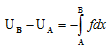In the absence of an external force, the sum total of kinetic and potential energies always remains constant.

?K + ?U = 0

Some other examples illustrating the concept of potential energy are given below:

(i) A wire supported by a rigid support and loaded at the other acquires potential energy.

(ii) A beam supported on two knife edges and loaded in the center acquires potential energy.

(iii) Water flowing in a river on a hill has potential energy stored in it. On falling down, potential energy is converted into electric energy in hydraulic dams.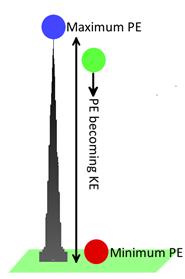Every substance which is in state of possessing potential energy has a tendency to lose it. That is why a wound up spring tends to open up. A stretched wire, a bent strip or a bent beam tends to acquire original configuration.

Thus, every system in equilibrium tends to exist in the state of minimum potential energy.

This basic fact has found a number of applications to explain the concept of a variety of phenomena in physics.

Here we shall discuss two cases:

(i) Potential energy of a body due to gravity, called gravitational potential energy.

(ii) Potential energy of a spring when it is elongated or compressed by an external force called elastic potential energy.

Gravitational Potential Energy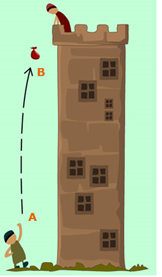It is the energy acquired by a body by virtue of its position in the gravitational field.

A body having mass ‘m’  always acted by a constant force F (= mg).  It is directed towards the earth. Here g is acceleration due to gravity.

Consider a body of mass m moving vertically downward from height y2 to y1, as shown in the Figure given below, the work done by this constant force of gravity is given by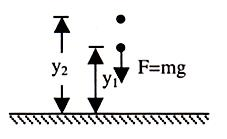W = -mg(y2-y1) = - (mgy2 - mgy1)

Here W depends on the difference the height or position.

So, we can define gravitational Potential Energy associated with the body as

U = mgy

Hence W = -(U2 - U1) = -ΔU

The negative sign implies that when gravity does positive work, the potential energy decreases. When gravity does negative work (the body moves upward), the potential energy increases.

We can observe that when a body falls from a height, it accelerates and increases its speed and hence gains K.E. This is at the expense of gravitational P.E. Hence we can relate potential energy and kinetic energy.

Relationship between Kinetic Energy and Potential Energy

We can relate P.E. and K.E. with the help of work energy theorem. Here the external force is gravitational force and the work done, W, by this force is equal to change in K.E. of the body. According Work Energy Theorem this W is equal to change in P.E. i.e.

W = ΔK = -ΔU    => ΔK = + ΔU = 0

Let the speed of body at height y1 and y2 be v1 and v2 respectively. (see Figure given below)

1/3mv22 - 1/2mv12 = -mg(y2 - y1)

1/2mv12 + mgy1  =  1/2mv22+ mgy1

Therefore the total energy, consists of K.E. and P.E., is conserved.

Now if the force is other than gravity and the work done by these forces is W', then according to Work-Energy theorem,

W = ΔK + ΔU

Elastic Potential Energy and Kinetic EnergyWhen a spring is elongated or compressed, work is done against the elastic restoring force of the spring (which we have discussed in previous illustration, see Figure given below). The work done is stored in the spring as elastic potential energy. Consider a mass 'm' attached to a spring. An applied force P stretches the spring by pulling the mass to the right. As shown  earlier for an applied force P which is proportional to x, the work done is (1/2)kx2. This amount of work done is called the elastic potential energy of the body.

The elastic restoring force acts in a direction opposite to the displacement. Hence, the worked one by the restoring force when the body moves x1 to x2 is

Wel = -(1/2)kx22 -(-(1/2)kx12)

= -(U2 - U1) = -ΔU

Let W' be the work done by the applied force P.

Total work done = change in kinetic energy.

W' + Wel = ΔK,

W' = ΔK + ΔU,

W' = (1/2mv22 - 1/2mv12) + (1/2Kx22 - 1/2Kx12)

W' = (1/2mv22 + 1/2mv12) - (1/2mv22+ 1/2Kx12)

As defined earlier, the sum of the kinetic and potential energies of the body is the total mechanical energy. Thus the work of all forces acting on the body with the exception of the elastic force equals the change in total mechanical energy of the body.

If there is no force other than the elastic restoring force acting on the body, then W' = 0, and the mechanical energy of the system is conserved.

1/2mv12 + 1/2kx12 = 1/2mv22 + 1/2kx22

Conservation of Energy

Law of conservation of energy was first stated by Robert Mayor in 1842. It states that,

”Energy can neither be created nor destroyed. It can be converted from one form to another. The sum total of energy, In this universe, is always same.”

Solved Problems

Problem 1:-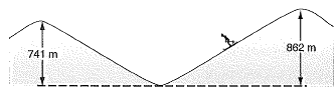Two snow – covered peaks are at elevations of 862 m and 741 m above the valley between them. A ski run extends from the top of the higher peak to the top of the lower one as shown in below figure. (a) A skier  starts from rest on the higher peak. At what speed would he arrive at the lower peak if he coasts without using the poles? Assume icy conditions, so that there is no friction. (b) After snow fall, a 54.4 kg skier making the same run also without using the poles only just makes it to the lower peak. By how much does the internal energy of her skies and the snow over which she traveled increase?

Concept:-

Kinetic energy K of a body is defined as,

K = ½ mv2

Here m is the mass of the body and v is the velocity of the body.

So, the initial kinetic energy Ki of the body having initial velocity vi will be,

Ki = ½ mvi2

And the final kinetic energy Kf of the body having final velocity vf will be,

Kf = ½ mvf2

Potential energy U is defined as,

U = mgh

Here m is the mass of the body, g is the free fall acceleration and h is the fall of height.

So, the initial potential energy Ui of the body having initial height yi will be,

Ui = mg yi

And the final potential energy Uf of the body having final height yf will be,

Uf = mg yf

Solution:-

(a) When the skier starts from rest on the higher peak, the initial velocity is zero results zero initial kinetic energy.

So, Ki = ½ mvi2

= ½ m(0)2

= 0

In accordance to law of conservation of energy, the sum of initial potential and kinetic energy (Ui + Ki) of the skier at the top of the peak is equal to the sum of final potential and kinetic energy (Uf + Kf)  of the skier at the bottom.

So, Ui + Ki = Uf + Kf

As Ki = 0, the above equation Ui + Ki = Uf + Kf will be,

Ui + Ki = Uf + Kf

So, Kf = Ui - Uf

Substitute ½ mvf2 for Kf, mg yi for Ui and mg yf for Uf in the equation Kf = Ui - Uf,

Kf = Ui - Uf

½ mvf2   = mg yi - mg yf

So, vf = √2 g (yi - yf)

To obtain the speed vf of the skier at the bottom, substitute 9.81 m/s2 for g, 862 m for yi and 741 m for yf in the equation vf = √2 g (yi - yf),

vf = √2 g (yi - yf)

= √2 (9.81 m/s2) (862 m - 741 m)

= 48.7 m/s

= (48.7 m/s) (10-3 km/1 m)

= (48.7 m/s) (10-3 km/1 m) (3600 s/1 h)

= 175.320 km/h

Rounding off to three significant figures, the speed at which the skier arrives at the lower peak would be 175 km/h.

(b) The increase in internal energy ΔE of her skis and the snow over which she travelled will be equal to difference of potential energy at the bottom and top (Ui - Uf).

So, ΔE = Ui - Uf

= mg yi - mg yf

= mg (yi – yf)

To obtain the increase in internal energy ΔE of her skis and the snow over which she travelled, substitute 54.4 kg for mass m, 9.81 m/s2 for g, 862 m for yi and 741 m for yf in the equation ΔE = mg (yi – yf),

ΔE  = mg (yi – yf)

= (54.4 kg) (9.81 m/s2) (862 m - 741 m)

= - 6.46×104 J

The negative sign signifies that, the internal energy of the snow and skis increased by 6.46×104 J.

From the above observation we conclude that, the increase in internal energy ΔE of her skis and the snow over which she travelled would be 6.46×104 J.

Problem 2:-

One end of a vertical spring is fastened to the ceiling. A weight is attached to the other end and slowly lowered to its equilibrium position. Show that the loss of gravitational potential energy of the weight equals one-half the gain in spring potential energy.

Concept:-

The potential energy (ΔUs) of a spring is defined as the,

ΔUs = ½ ky2      …… (1)

Where k is the spring constant and y is the extension of the spring from its original position.

Gravitational potential energy (ΔUg) of the weight (mg) would be,

ΔUg = -mgy        …… (2)

Solution:-

Force acting (F) on the spring due to an extension y will be,

F = ky                …… (3)

Where, k is the spring constant.

Weight is equal (mg) to the force due to extension of the spring (F).

So, F = mg     …… (4)

From equation (3) and (4), we get,

F = ky = mg     …… (5)

From equation (1), we get,

ΔUs = ½ ky2

= ½ (ky) (y)     …… (6)

To obtain ΔUs, substitute mg for ky in the equation, ΔUs= ½ (ky) (y),

ΔUs= ½ (ky) (y)

= ½ mgy

So, 2 ΔUs = mgy

= - (-mgy)

= - ΔUg          (Since, ΔUg = -mgy)    …… (7)

From equation (7) we observed that, the loss of gravitational potential energy of the weight equals one-half the gain in spring potential energy.

Problem 3:-

A very small ice cube is released  from the edge of a hemispherical frictionless bowl whose radius is 23.6 cm as shown in below figure. How fast is the cube moving at the bottom of the bowl?Concept:-

In an isolated system in which only conservative forces act, the total mechanical energy remains constant. That is, the initial value of the total mechanical energy (Ki +Ui)  is equal to the final value (Kf +Uf). Here Ki is the initial kinetic energy, Ui is the initial potential energy, Kf is the final kinetic energy and Uf is the final potential energy.

So, Kf +Uf = Ki +Ui

½ mvf2 + mgyf = ½ mvi2 + mgyi

Solution:-

Since the ice cube is released from the edge of a hemispherical frictionless bowl, so we have to substitute –r for yf (negative sign due to down ward direction) , 0 for vi (at the point of release) and 0 for yi (at the point of release) in the equation ½ mvf2 + mgyf = ½ mvi2 + mgyi.

½ mvf2 + mgyf = ½ mvi2 + mgyi

½ vf2 + gyf = ½ vi2 + gyi

½ vf2 + g(-r) = ½ (0)2 + g(0)

½ vf2 = gr

So, the final velocity vf of the cube at the bottom of the bowl would be,

vf = √2gr

To obtain the final velocity vf, substitute 9.81 m/s2 and 23.6 cm for r in the equation vf = √2gr,

vf = √2gr

= √2(9.81 m/s2) (23.6 cm)

= √2(9.81 m/s2) (23.6 cm×10-2 m/1 cm)

= 2.15 m/s

From the above observation we conclude that, the speed of the cube at the bottom of the bowl would be 2.15 m/s.

Related Resources

Look into the Sample Papers of Previous Years to get a hint of the kinds of questions asked in the exam.

You can get the knowledge of Useful Books of Physics.

```### Course Features

• 101 Video Lectures
• Revision Notes
• Previous Year Papers
• Mind Map
• Study Planner
• NCERT Solutions
• Discussion Forum
• Test paper with Video Solution